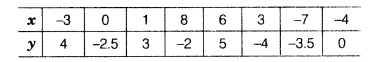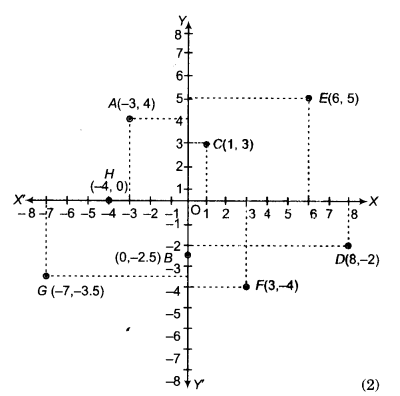# Plot the points (x, y) given in the following table on the plane choosing suitable units

Plot the points (x, y) given in the following table on the plane choosing suitable units of distances on the axes.The given points are A(-3,4),S(0,-2 5),C(1,3), D(8,-2), E(6,5), F(3,-4), G(-7,-3.5)and H(-4,0).
Now, we plot the points on the graph paper as shown by dots in the figure below